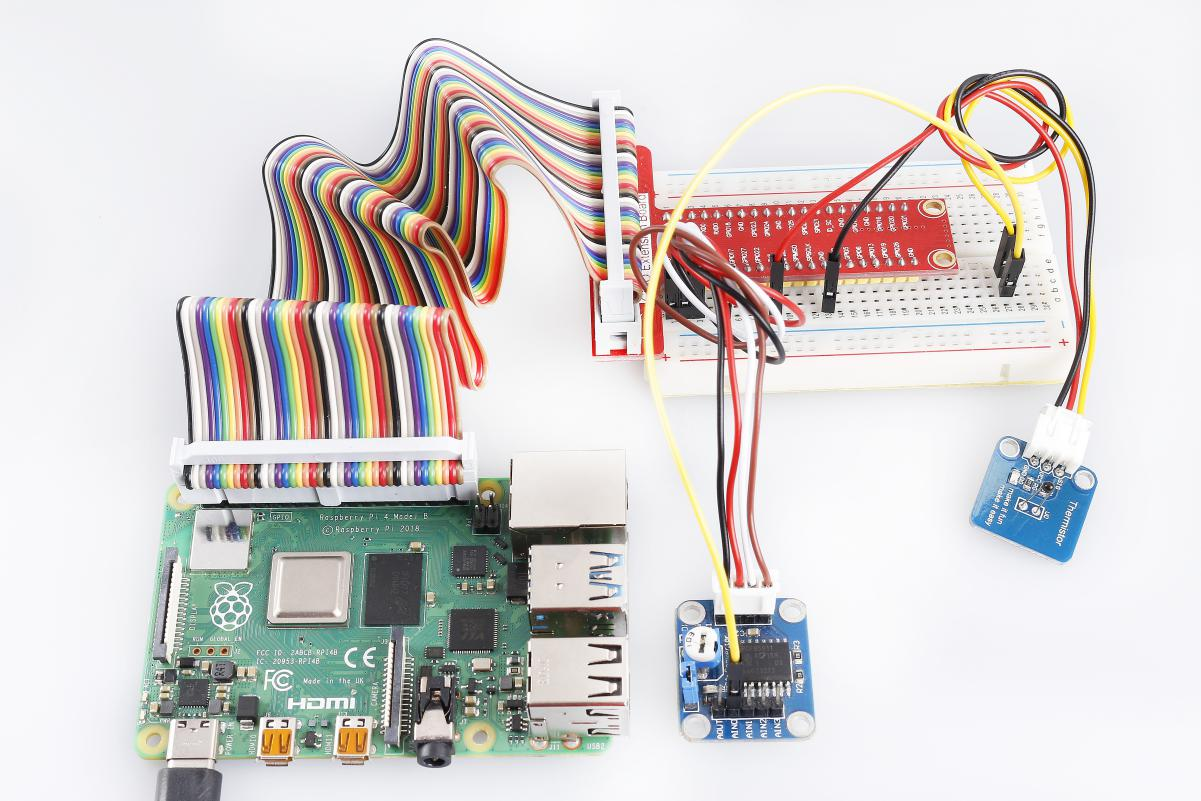# Lesson 18 Temperature Sensor¶

Introduction

A temperature sensor is a component that senses temperature and converts it into output signals. By material and component features, temperature sensors can be divided into two types: thermal resistor and thermocouple. Thermistor is one kind of the former type. It is made of semiconductor materials; most thermistors are negative temperature coefficient (NTC) ones, the resistance of which decreases with rising temperature. Since their resistance changes acutely with temperature changes, thermistors are the most sensitive temperature sensors.

There are two kinds of thermistor module in this kit (as shown below).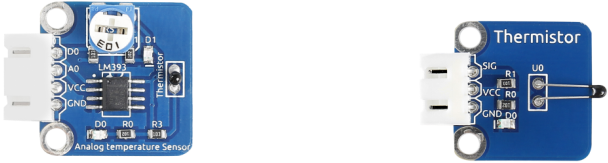Required Components

• 1 * Raspberry Pi

• 1 * Breadboard

• 1 * Analog-temperature Sensor module

• 1 * Thermistor module

• 1 * PCF8591

• 1 * 3-Pin anti-reverse cable

• 1 * 4-Pin anti-reverse cable

• Several Jumper wires

Experimental Principle

This module is based on the principle of the thermistor, whose resistance varies significantly with ambient temperature. When the ambient temperature increases, the resistance of the thermistor decreases; when decreases, it increases. It can detect surrounding temperature changes in a real-time manner.

In this experiment, we use an analog-digital converter PCF8591 to convert analog signals into digital ones.

The schematic diagram for analog temperature sensor: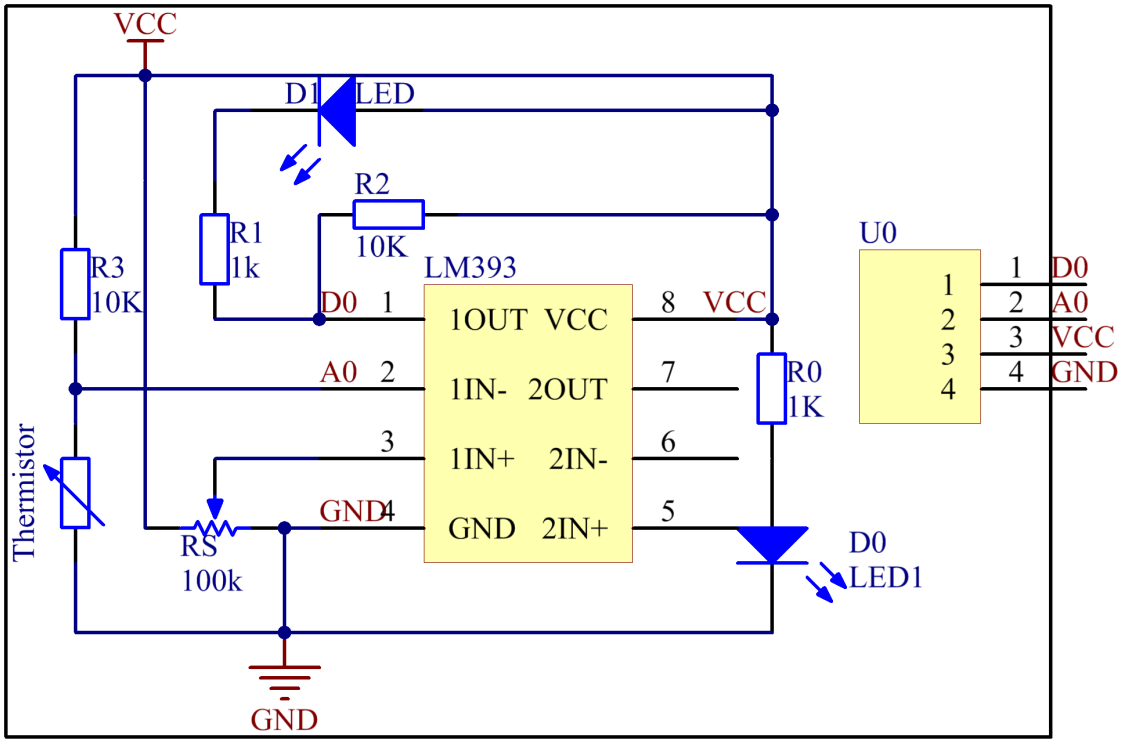The schematic diagram for the thermistor module: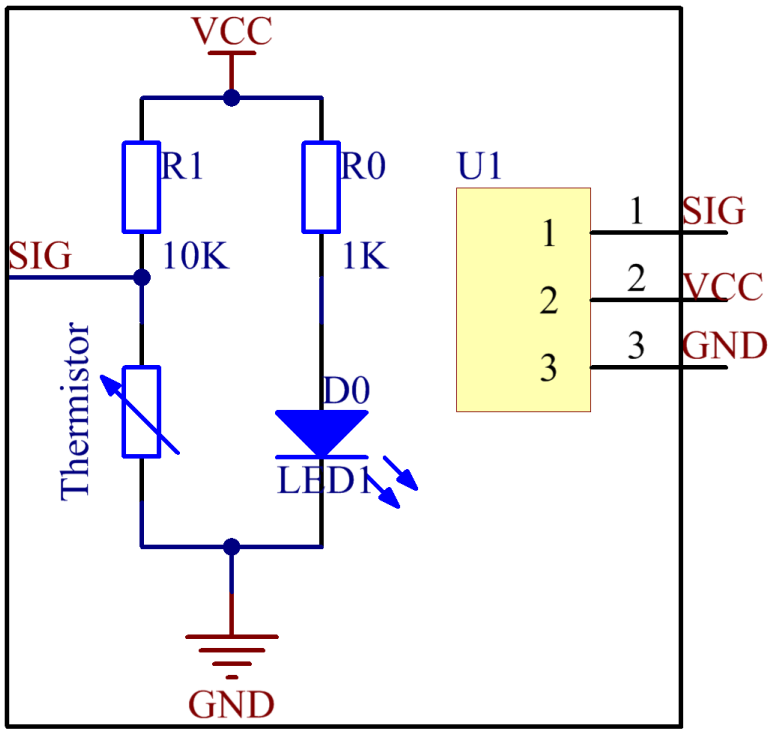Experimental Procedures

Step 1: Build the circuit.

 Raspberry Pi GPIO Extension Board PCF8591 Module SDA SDA1 SDA SCL SCL1 SCL 3.3V 3V3 VCC GND GND GND

For thermistor module:

 Thermistor Module GPIO Extension Board PCF8591 Module SIG * AIN0 VCC 3V3 VCC GND GND GND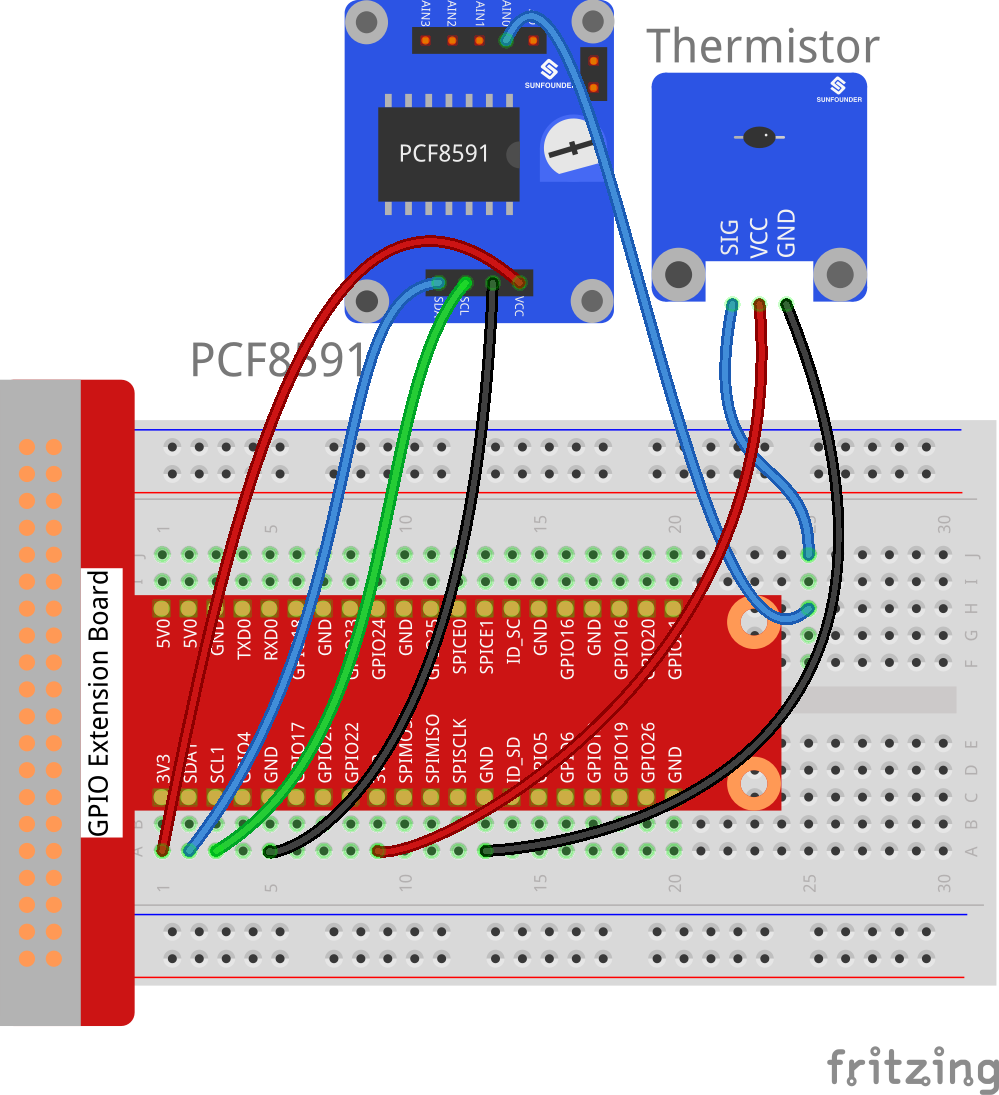For analog temperature sensor module：

 Analog Temperature Module GPIO Extension Board PCF8591 Module DO GPIO17 * AO * AIN0 VCC 3V3 VCC GND GND GND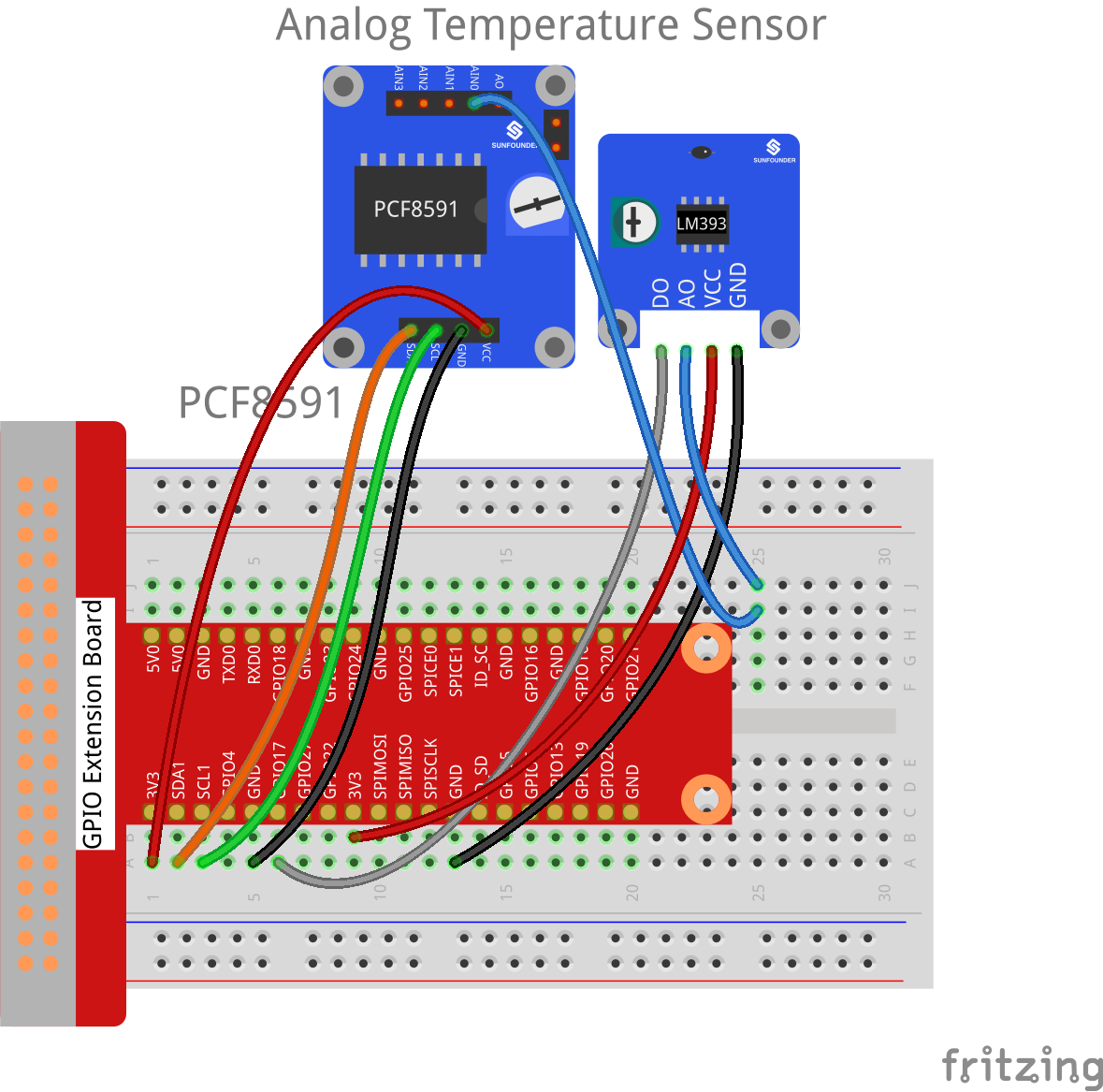For C Users:

Step 2: Change directory.

```cd /home/pi/SunFounder_SensorKit_for_RPi2/C/18_thermistor/
```

Step 3: Compile.

```gcc thermistor.c -lwiringPi -lm
```

Note

If it does not work after running, or there is an error prompt `wiringPi.h: No such file or directory`, please refer to WiringPi to install it.

Step 4: Run.

```sudo ./a.out
```

Code

```#include <stdio.h>
#include <wiringPi.h>
#include <pcf8591.h>
#include <math.h>

#define             PCF     120
#define             DOpin   0

void Print(int x)
{
switch(x)
{
case 0:
printf("\n************\n"  );
printf(  "* Too Hot! *\n"  );
printf(  "************\n\n");
break;
case 1:
printf("\n***********\n"  );
printf(  "* Better~ *\n"  );
printf(  "***********\n\n");
break;
default:
printf("\n**********************\n"  );
printf(  "* Print value error. *\n"  );
printf(  "**********************\n\n");
break;
}
}

int main()
{
unsigned char analogVal;
double Vr, Rt, temp;
int tmp, status;

if(wiringPiSetup() == -1){
printf("setup wiringPi failed !");
return 1;
}
// Setup pcf8591 on base pin 120, and address 0x48
pcf8591Setup(PCF, 0x48);

pinMode(DOpin, INPUT);

status = 0;
while(1) // loop forever
{
printf("loop");
analogVal = analogRead(PCF + 0);
Vr = 5 * (double)(analogVal) / 255;
Rt = 10000 * (double)(Vr) / (5 - (double)(Vr));
temp = 1 / (((log(Rt/10000)) / 3950)+(1 / (273.15 + 25)));
temp = temp - 273.15;
printf("Current temperature : %lf\n", temp);

// For a threshold, uncomment one of the code for
// which module you use. DONOT UNCOMMENT BOTH!
//---------------------------------------------
// 1. For Analog Temperature module(with DO)

// 2. For Thermister module(with sig pin)
// if (temp > 33) tmp = 0;
// else if (temp < 31) tmp = 1;
//---------------------------------------------

if (tmp != status)
{
Print(tmp);
status = tmp;
}

delay (200);
}
return 0;
}
```

For Python Users:

Step 2: Change directory.

```cd /home/pi/SunFounder_SensorKit_for_RPi2/Python/
```

Step 3: Run.

```sudo python3 18_thermistor.py
```

Code

```#!/usr/bin/env python3
import PCF8591 as ADC
import RPi.GPIO as GPIO
import time
import math

DO = 17
GPIO.setmode(GPIO.BCM)

def setup():
GPIO.setup(DO, GPIO.IN)

def Print(x):
if x == 1:
print ('')
print ('***********')
print ('* Better~ *')
print ('***********')
print ('')
if x == 0:
print ('')
print ('************')
print ('* Too Hot! *')
print ('************')
print ('')

def loop():
status = 1
tmp = 1
while True:
Vr = 5 * float(analogVal) / 255
Rt = 10000 * Vr / (5 - Vr)
temp = 1/(((math.log(Rt / 10000)) / 3950) + (1 / (273.15+25)))
temp = temp - 273.15
print ('temperature = ', temp, 'C')

# For a threshold, uncomment one of the code for
# which module you use. DONOT UNCOMMENT BOTH!
#################################################
# 1. For Analog Temperature module(with DO)
tmp = GPIO.input(DO)
#
# 2. For Thermister module(with sig pin)
#if temp > 33:
#   tmp = 0
#elif temp < 31:
#   tmp = 1
#################################################

if tmp != status:
Print(tmp)
status = tmp

time.sleep(0.2)

if __name__ == '__main__':
try:
setup()
loop()
except KeyboardInterrupt:
pass
```

Now touch the thermistor and you can see the value of current temperature printed on the screen change accordingly.

Temperature alarm setting:

If you use the Analog Temperature Sensor module, uncomment the line under 1:

For C language:

```// For a threshold, uncomment one of the code for
// which module you use. DONOT UNCOMMENT BOTH!
//-----------------------------------------
// 1. For Analog Temperature module(with DO)

// 2. For Thermister module(with sig pin)
// if (temp > 33) tmp = 0;
// else if (temp < 31) tmp = 1;
```

For Python

```#################################################
# 1. For Analog Temperature module(with DO)
tmp = GPIO.input(DO);

# 2. For Thermister module(with sig pin)
#if temp > 33:
# tmp = 0;
#elif temp < 31:
# tmp = 1;
#################################################
```

If you use the Thermistor module, uncomment the lIne under 2:

For C language:

```// For a threshold, uncomment one of the code for
// which module you use. DONOT UNCOMMENT BOTH!
//-------------------------------------------
// 1. For Analog Temperature module(with DO)
// tmp = digitalRead(DO);

// 2. For Thermister module(with sig pin)
if (temp > 33) tmp = 0;
else if (temp < 31) tmp = 1;
//------------------------------------------
```

For Python

```#################################################
# 1. For Analog Temperature module(with DO)
#tmp = GPIO.input(DO);
#
# 2. For Thermister module(with sig pin)
if temp > 33:
tmp = 0;
elif temp < 31:
tmp = 1;
#################################################
```

After editing the code, repeat step 2, 3, and 4 (or step 2, 3 for Python users).

You can still see temperature value printed on the screen constantly. If you pinch the thermistor for a while, its temperature will rise slowly. “Too Hot!” will be printed on the screen. Release your fingers, and let it stay in the open air for a while, or blow on the module. When the temperature drops down slowly, “Better” will be printed.

Note

The analog temperature sensor adjusts alarm temperature by the potentiometer on the module. The thermistor changes the alarm temperature by program.

The physical picture for analog temperature sensor: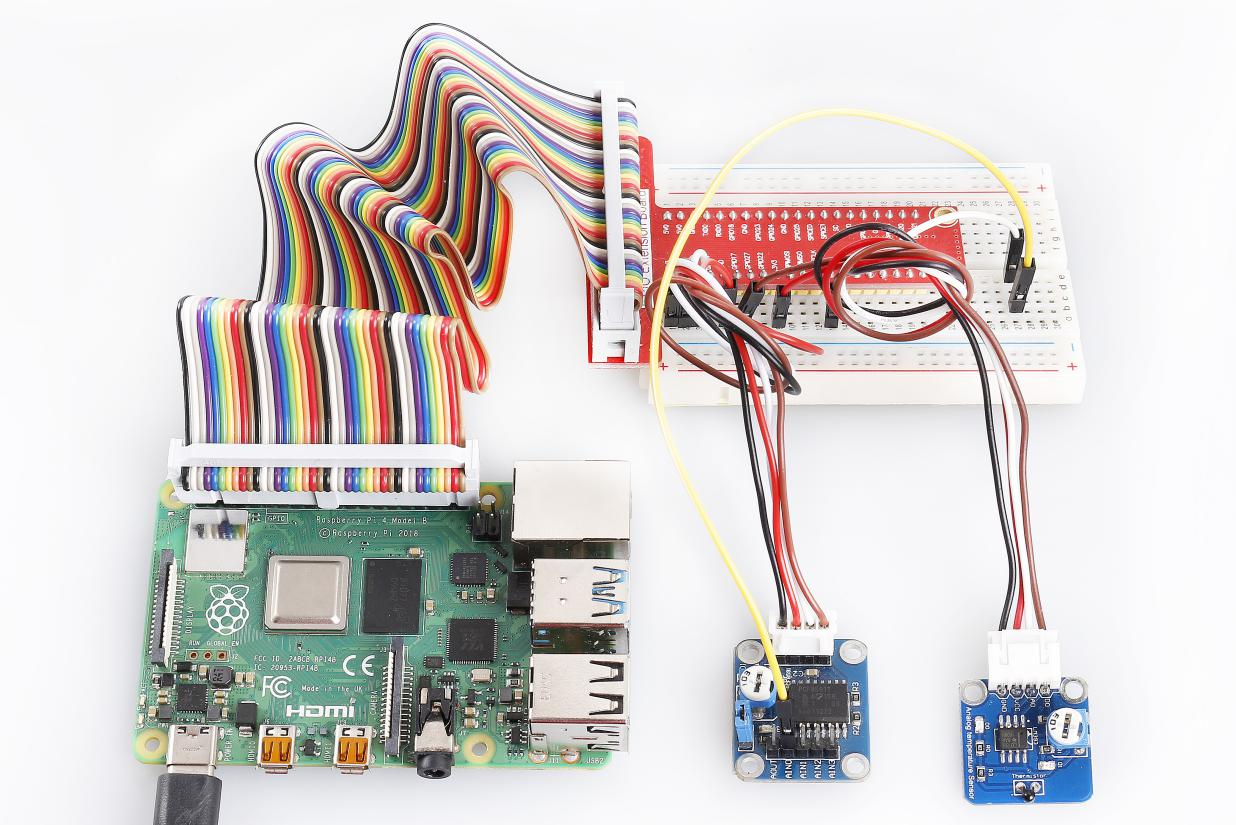The physical picture for thermistor module: